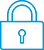cancel
Showing results for
Search instead for
Did you mean:It has been a while since anyone has replied. Simply ask a new question if you would like to start the discussion again.Level 1
4 2 1 0
Message 1 of 5
1,151
Flag Post

Solved!

# Using SOLVE on VectorsHP Recommended
HP Prime

After seeing the RETURN function on how to Return multiple functions of a single variable as a vector, how can I use the SOLVE function on this vector.

Let´s say I made a program that returns VECTOR(x) expressed as [a(x),b(x),c(x)]
Assuming at any given time that I know the value of a(x), b(x) or c(x), what would be the sintaxis for solving VECTOR(x) for x?

Tags (1)
4 REPLIES 4Level 6
305 297 32 76
Message 2 of 5
Flag PostHP Recommended

Do I understand you well that you want to solve:

VECTOR(x)=[0,0,0] ?

That would mean that

a(x)=0

b(x)=0

c(x)=0

These are 3 equations with 1 unknown, which has generally no solution.

For example,

When

a(x)=x+3

b(x)=x+5

c(x)=x+9

There is no such solution.Level 8
622 609 117 207
Message 3 of 5
Flag PostHP Recommended

For those following this thread, please note that this question was asked and answered here: http://www.hpmuseum.org/forum/thread-10807-post-98343.html#pid98343

-Joe-Level 1
4 2 1 0
Message 4 of 5
Flag PostHP Recommended

Thanks Joe!

That was actually me. Here´s the final code. The solution was using a list instead of a vector.

Update 6-1-2018 included warning messages for low and high flows.

EXPORT PUMP1(X)
BEGIN

LOCAL h,a;

h:=-3.96912716744ᴇ−6*X^3+1.33502710425ᴇ−3*X^2-0.230829109114*X+187.74099966;

LOCAL Eff;

Eff:=8.58164056499ᴇ−8*X^3-1.00216261706ᴇ−3*X^2+0.480844232375*X-0.029541916438;

LOCAL PWR;

PWR:=0.25415412597*X+68.328377716;

LOCAL NPSH;

NPSH:=3.42624923327ᴇ−7*X^3-1.02340333129ᴇ−4*X^2+7.55229835063ᴇ−3*X+2.60628404048;

a:={{"h(m)",h},{"Eff(%)",Eff},{"SHP(kW)",PWR},{"NPSH(m)=",NPSH}};

IF X<80 THEN RETURN {" Warning: Flow below minimum",a};END;

IF X>340 THEN RETURN {"Warning: Flow above end of curve",a};END;

PRINT("h(m)");PRINT(h);

PRINT("Eff(%)");PRINT(Eff);

PRINT("SHP(kW");PRINT(PWR);

PRINT("NPSH(m)");PRINT(NPSH);

RETURN a;

END;

Tags (1)Level 1
4 2 1 0
Message 5 of 5
Flag PostHP Recommended

I found another approach which is copying the 2 variable statistics app and renaming it after the Pump.

For the app follow the link

http://www.hpmuseum.org/forum/thread-10807-post-98863.html#pid98863

† The opinions expressed above are the personal opinions of the authors, not of HP. By using this site, you accept the Terms of Use and Rules of Participation